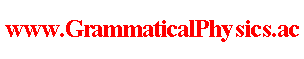since 2006 Help　Sitemap < Forum > < Definition > < Abstracts for the Meetings Held by the Physical Society > < JPS 2009 Spring Meeting > 30pSF-8Energy Ambiguity Problem of New Quantum GrammarUda School / Yuuichi Uda---If E＝p^2/(2m), a functional Φ defined as Φ[χ]＝exp{(i/h)α∫dt[pχ(t)－Et]} is a formal solution of the new grammar version of Schrodinger equation(JPS 2007 Spring Meeting 28pSL-11) on the condition V＝0, and represents the same quantum history as one represented by a time dependent wave function φ defined as φ(x,t)＝exp[(i/h)(px－Et)] of existing quantum mechanics.Seeing this Φ well, I wonder if E does not work at all.For I think that we may use ∫dt Et＝0 by calculating ∫dt Et first because Et is an odd function of t.Moreover, I wonder if we may think that Φ defined as Φ[χ]＝exp[(i/h)α∫dt pχ(t)] represents the same quantum history as represented by Φ defined as Φ[χ]＝exp{(i/h)α∫dt[pχ(t)－f(t)]} not only for the case ∫dt f(t)＝0 but also for an arbitrary function f because they differs from each other only by the phase factor.If so, it is a problem that above Φ may be a solution of the new grammar version of Schrodinger equation and may not be so at the same time and it is a problem that in case explicit time dependence of a wave function in the existing quantum mechanics represents some physical situation my new grammar can not represent the situation.Is it really so?I noticed that it is not necessarily so.For the domain of definition of Φ including E term is different from the domain of definition of Φ without E term.∫dt[pχ(t)-Et]} must be finite for χ in the domain of definition of Φ defined as Φ[χ]＝exp{(i／h)α∫dt[pχ(t)-Et]}.Such χ can be represented as χ(t)＝f(t)＋(E／p)t using f such as ∫dt f(t) is finite.On the other hand, ∫dtχ(t) must be finite for χ in the domain of definition of Φ defined as Φ[χ]＝exp[(i／h)α∫dt pχ(t)].Therefore, these two domains of definition are not only different from each other but also do not have a common part at all.How about understanding that Φ defined as Φ[χ]＝exp[α∫dt pχ(t)] is different from Φ defined as Φ[χ]＝exp{α∫dt[pχ(t)－Et]} because their domains of definition are different?For a general functional Φ defined as Φ[χ]＝exp{(i／h)α∫dt[pχ(t)-f(t)]}, too, we can possibly say that f which changes the domain of definition is not negligible.The idea is hopeful if we can improve the argument at law level such as the proof of the new grammar version of Ehrenfest theorem to a more rigorous form by taking the domain of definition into account. --- This article is a rewrite of the following article. JPS 2009 Spring Meeting@Abstracts for the Meetings Held by the Physical Society@Definition of Grammatical Physics@Grammatical Physics@Forum@Vintage(2008-2014) Author Yuichi Uda, Write start at 2015/05/23/19:37JST, Last edit at 2015/05/23/19:37JST